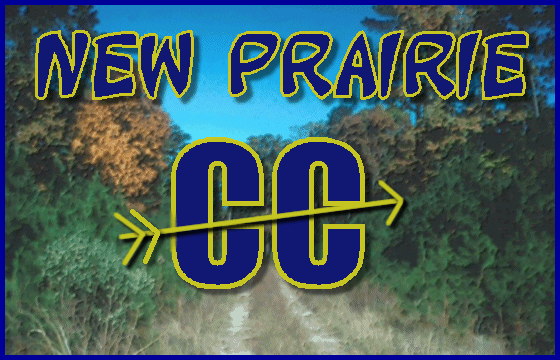New Prairie Cross CountryNP 2022 Invite Results
````````````````````````````````````````````````````````````````````````````````

NP 2021 Invite Results
````````````````````````````````````````````````````````````````````````````````
NP 2020 Invite Results
````````````````````````````````````````````````````````````````````````````````
NP 2019 Invite Results

Boys Reserve Overall 2019

````````````````````````````````````````````````````````````````````````````````

````````````````````````````````````````````````````````````````````````````````

NP 2018 Invite Results

Boys Reserve Overall 2018

````````````````````````````````````````````````````````````````````````````````

````````````````````````````````````````````````````````````````````````````````

NP 2017 Invite Results

Boys Reserve Overall 2017

````````````````````````````````````````````````````````````````````````````````

````````````````````````````````````````````````````````````````````````````````

NP 2016 Invite Results

Boys Reserve Overall 2016

````````````````````````````````````````````````````````````````````````````````

````````````````````````````````````````````````````````````````````````````````

NP 2015 Invite Results

Boys Reserve Overall 2015

````````````````````````````````````````````````````````````````````````````````

````````````````````````````````````````````````````````````````````````````````
NP 2014 Invite Results

Boys Reserve Overall 2014

````````````````````````````````````````````````````````````````````````````````

````````````````````````````````````````````````````````````````````````````````
NP 2013 Invite Results

Boys Reserve Overall 2013

````````````````````````````````````````````````````````````````````````````````

````````````````````````````````````````````````````````````````````````````````

Boys Reserve Overall 2012

````````````````````````````````````````````````````````````````````````````````

````````````````````````````````````````````````````````````````````````````````
Corrected NP 2011 Invite Results

Boys Reserve Overall 2011

````````````````````````````````````````````````````````````````````````````````

Boys Reserve Team 2011

````````````````````````````````````````````````````````````````````````````````
Corrected NP 2010 Invite Results

Boys Reserve Overall 2010 (cancelled due to weather)

````````````````````````````````````````````````````````````````````````````````

Boys Reserve Team 2010 (cancelled due to weather)

````````````````````````````````````````````````````````````````````````````````

Boys Reserve Overall 2009

````````````````````````````````````````````````````````````````````````````````

Boys Reserve Team 2009

````````````````````````````````````````````````````````````````````````````````

Boys Reserve 2008

````````````````````````````````````````````````````````````````````````````````

Boys Reserve 2007

````````````````````````````````````````````````````````````````````````````````

Boys Reserve 2006

````````````````````````````````````````````````````````````````````````````````